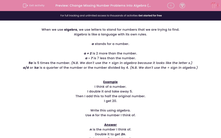# Change Missing Number Problems into Algebra (2)

In this worksheet, students express missing number problems algebraically.This content is premium and exclusive to EdPlace subscribers.Key stage:  KS 2

Curriculum topic:   Maths and Numerical Reasoning

Curriculum subtopic:   Equations and Algebra

Difficulty level:#### Worksheet Overview

When we use algebra, we use letters to stand for numbers that we are trying to find.

Algebra is like a language with its own rules.

a stands for a number.

a + 2 is 2 more than the number.

a - 7 is 7 less than the number.

5a is 5 times the number. (N.B. We don't use the × sign in algebra because it looks like the letter x.)

a/4 or ¼a is a quarter of the number or the number divided by 4. (N.B. We don't use the ÷ sign in algebra.)

Example

I think of a number.

I double it and take away 5.

Then I add this to half the original number.

I get 20.

Write this using algebra.

Use n for the number I think of.

n is the number I think of.

Double it to get 2n.

Take away 5 to get 2n - 5.

Then add this to ½n to give 2n - 5 + ½n .

This equals 20.

So we write:

2n - 5 + ½n = 20

### What is EdPlace?

We're your National Curriculum aligned online education content provider helping each child succeed in English, maths and science from year 1 to GCSE. With an EdPlace account you’ll be able to track and measure progress, helping each child achieve their best. We build confidence and attainment by personalising each child’s learning at a level that suits them.

Get started